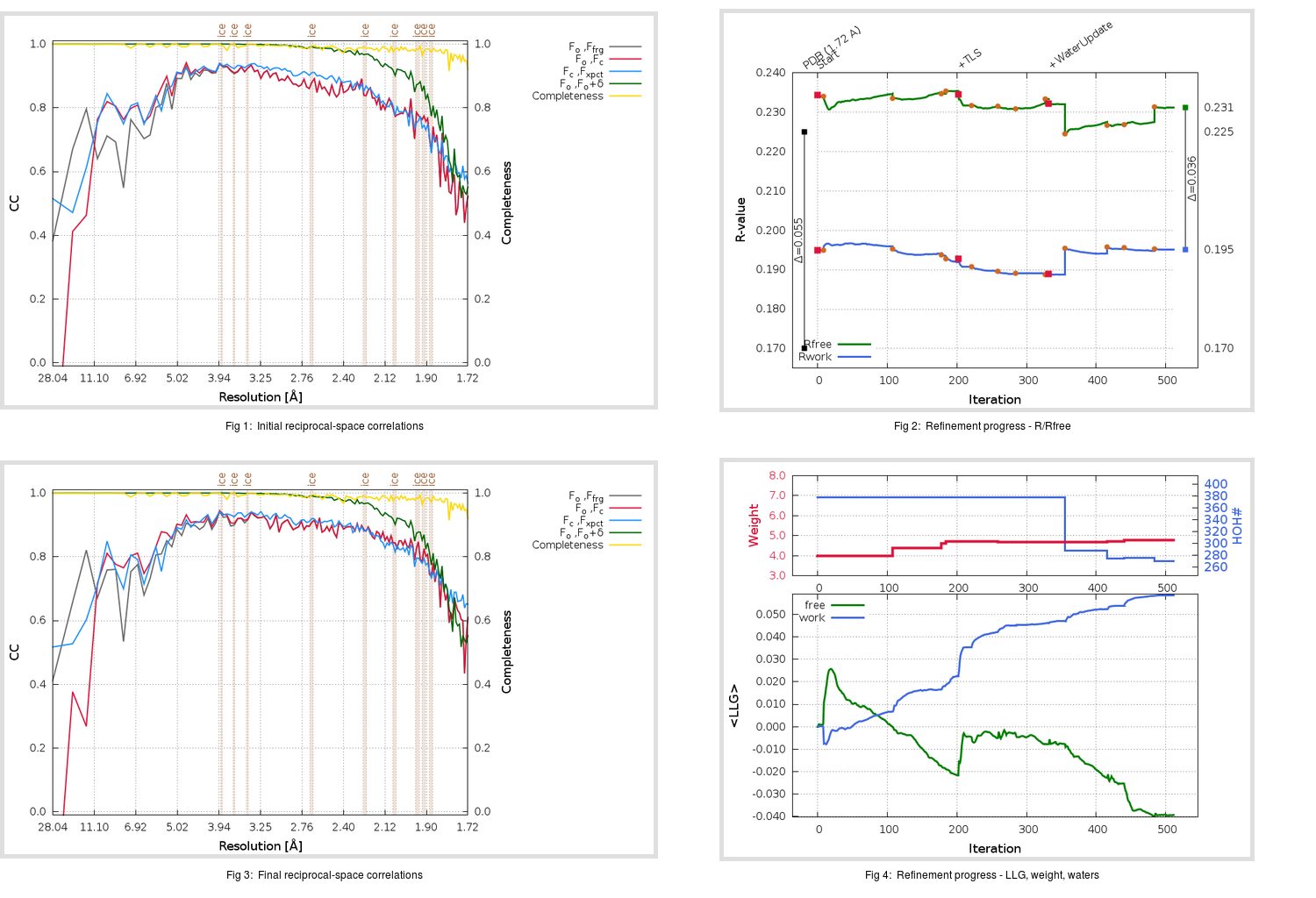Content:

## Deposited

` `
 Date deposited Date data collection Resolution R, Rfree 20200315 20200226 1.72 0.1670 0.2250

Molprobity (CCP4 7.0 version) summary:

```Ramachandran outliers =   0.33 %
favored =  97.68 %
Rotamer outliers      =   3.57 %
C-beta deviations     =     1
Clashscore            =   8.55
RMS(bonds)            =   0.0131
RMS(angles)           =   1.82
MolProbity score      =   1.95
Resolution            =   1.72
R-work                =   0.1670
R-free                =   0.2250
```

```Number of waters      =   377

<B> (all atoms) =   32.04 ( sd =   11.16 ) for       2911 non-hydrogen atoms
<B>   (protein) =   29.57 ( sd =    8.75 ) for       2496 non-hydrogen atoms
<B>     (water) =   45.57 ( sd =   11.74 ) for        377 non-hydrogen atoms
<B>    (others) =   45.54 ( sd =   18.46 ) for         38 non-hydrogen atoms

B min/max       (all non-hydrogen atoms) =   17.14 /   86.68
B min/max   (protein non-hydrogen atoms) =   17.14 /   86.68
B min/max     (water non-hydrogen atoms) =   20.55 /   82.96
B min/max     (other non-hydrogen atoms) =   33.26 /   78.15
```

## BUSTER (re-)refinement

` `

Molprobity (CCP4 7.0 version) summary:

```Ramachandran outliers =   0.33 %
favored =  98.68 %
Rotamer outliers      =   0.71 %
C-beta deviations     =     0
Clashscore            =   6.56
RMS(bonds)            =   0.0121
RMS(angles)           =   1.60
MolProbity score      =   1.36
Resolution            =   1.72
R-work                =   0.1951
R-free                =   0.2311
```

```Number of waters      =   270

<B> (all atoms) =   31.50 ( sd =    9.99 ) for       2804 non-hydrogen atoms
<B>   (protein) =   29.62 ( sd =    7.85 ) for       2496 non-hydrogen atoms
<B>     (water) =   45.60 ( sd =   11.56 ) for        270 non-hydrogen atoms
<B>    (others) =   43.69 ( sd =   18.08 ) for         38 non-hydrogen atoms

B min/max       (all non-hydrogen atoms) =   12.67 /   92.86
B min/max   (protein non-hydrogen atoms) =   12.67 /   63.17
B min/max     (water non-hydrogen atoms) =   22.32 /   92.86
B min/max     (other non-hydrogen atoms) =   33.19 /   67.11
```

Refinement progression:Results:

` `
 File Remark 5RE9_aB_refine.01_03_refine.pdb.gz exact refinement commands are in header 5RE9_aB_refine.01_03_refine.mtz.gz including original deposited data and several re-refinement map coefficients 5RE9_aB_refine.01_03_BUSTER_model.cif.gz including any non-standard compound restraints 5RE9_aB_refine.01_03_BUSTER_refln.cif.gz